# Working with Worksheet Tables

Tables in Excel are a way of grouping a range of cells into a single entity that has common formatting or that can be referenced from formulas. Tables can have column headers, autofilters, total rows, column formulas and default formatting.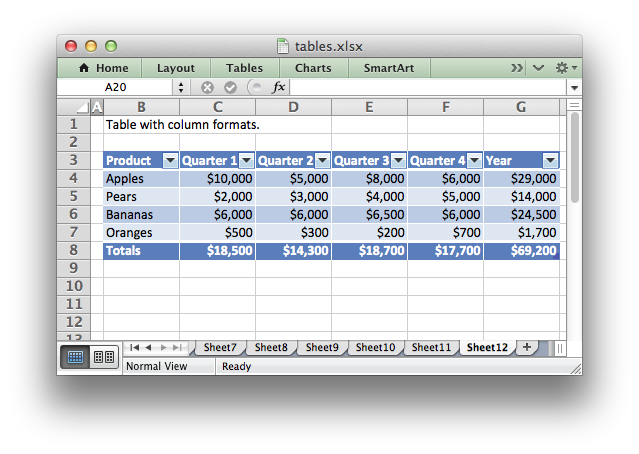For more information see An Overview of Excel Tables in the Microsoft Office documentation.

Note

Tables aren’t available in XlsxWriter when `Workbook()` `'constant_memory'` mode is enabled.

Tables are added to a worksheet using the `add_table()` method:

```worksheet.add_table('B3:F7', {options})
```

The data range can be specified in ‘A1’ or ‘Row/Column’ notation (see Working with Cell Notation):

```worksheet.add_table('B3:F7')
# Same as:
```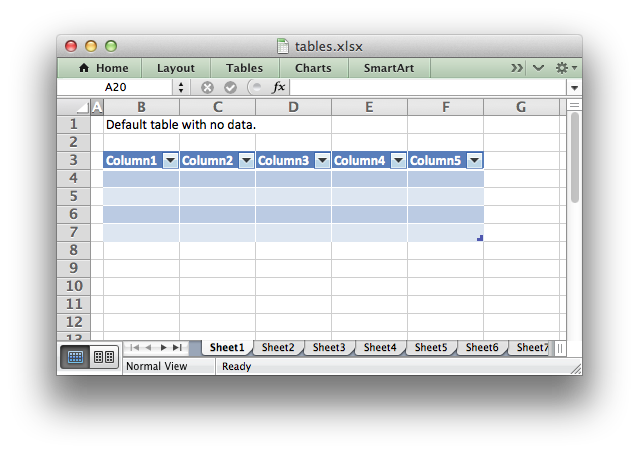The options parameter should be a dict containing the parameters that describe the table options and data. The available options are:

 data autofilter header_row banded_columns banded_rows first_column last_column style total_row columns name

These options are explained below. There are no required parameters and the options parameter is itself optional if no options are specified (as shown above).

## data

The `data` parameter can be used to specify the data in the cells of the table:

```data = [
['Apples', 10000, 5000, 8000, 6000],
['Pears',   2000, 3000, 4000, 5000],
['Bananas', 6000, 6000, 6500, 6000],
['Oranges',  500,  300,  200,  700],

]

```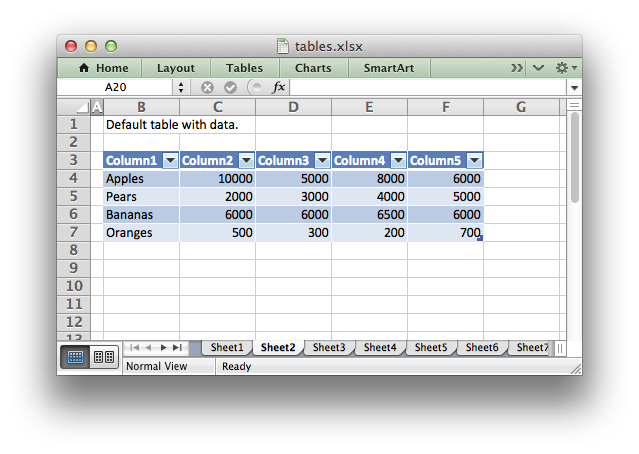Table data can also be written separately, as an array or individual cells:

```# These statements are the same as the single statement above.
worksheet.write_row('B4', data)
worksheet.write_row('B5', data)
worksheet.write_row('B6', data)
worksheet.write_row('B7', data)
```

Writing the cell data separately is occasionally required when you need to control the `write_()` methods used to populate the cells or if you wish to modify individual cell formatting.

The `data` structure should be an list of lists holding row data as shown above.

The `header_row` parameter can be used to turn on or off the header row in the table. It is on by default:

```# Turn off the header row.
```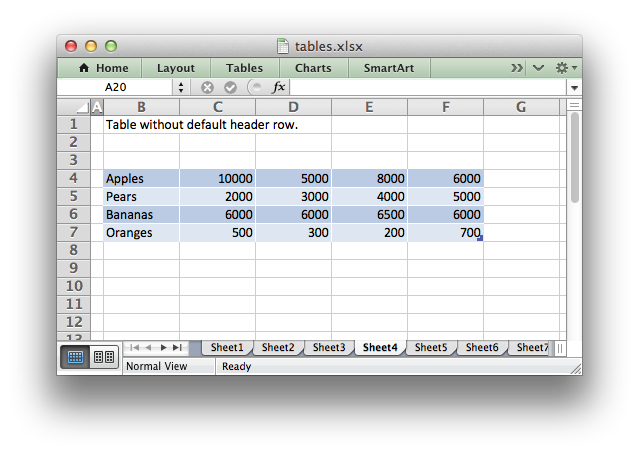The header row will contain default captions such as `Column 1`, `Column 2`, etc. These captions can be overridden using the `columns` parameter below.

## autofilter

The `autofilter` parameter can be used to turn on or off the autofilter in the header row. It is on by default:

```# Turn off the default autofilter.
```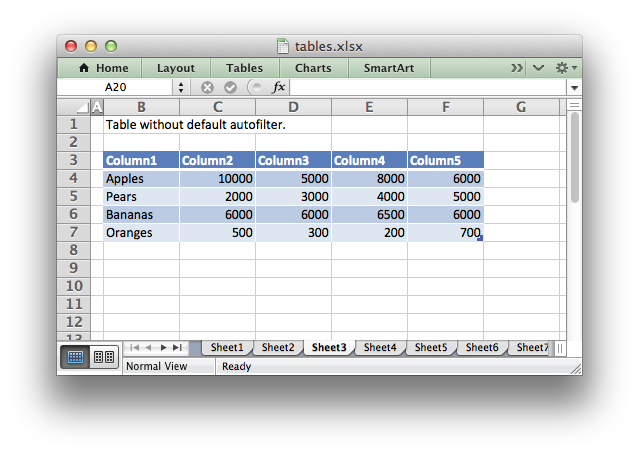The `autofilter` is only shown if the `header_row` is on. Filter conditions within the table are not supported.

## banded_rows

The `banded_rows` parameter can be used to create rows of alternating color in the table. It is on by default:

```# Turn off banded rows.
```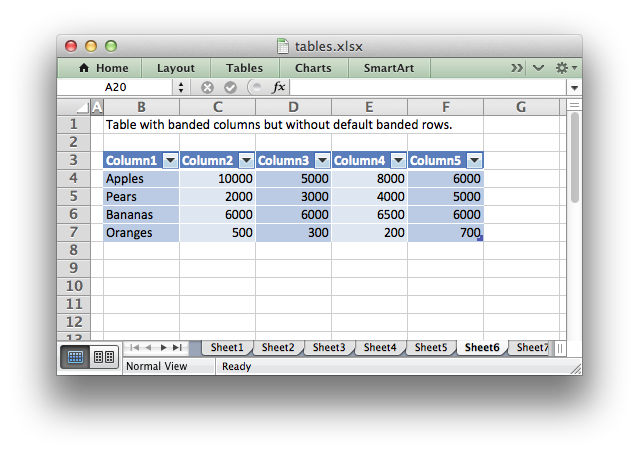## banded_columns

The `banded_columns` parameter can be used to used to create columns of alternating color in the table. It is off by default:

```# Turn on banded columns.
```

See the above image.

## first_column

The `first_column` parameter can be used to highlight the first column of the table. The type of highlighting will depend on the `style` of the table. It may be bold text or a different color. It is off by default:

```# Turn on highlighting for the first column in the table.
```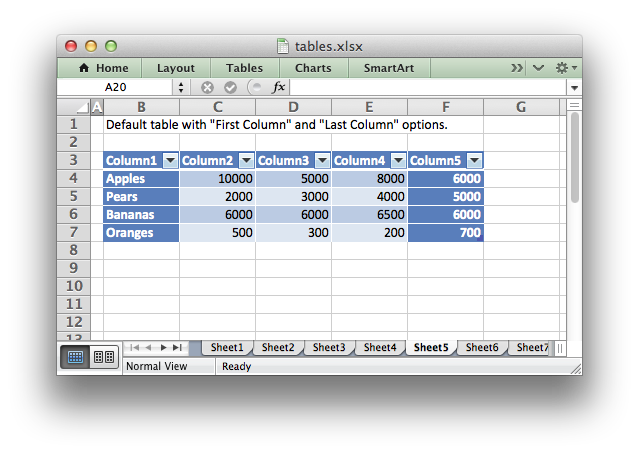## last_column

The `last_column` parameter can be used to highlight the last column of the table. The type of highlighting will depend on the `style` of the table. It may be bold text or a different color. It is off by default:

```# Turn on highlighting for the last column in the table.
```

See the above image.

## style

The `style` parameter can be used to set the style of the table. Standard Excel table format names should be used (with matching capitalization):

```worksheet.add_table('B3:F7', {'data': data,
'style': 'Table Style Light 11'})
```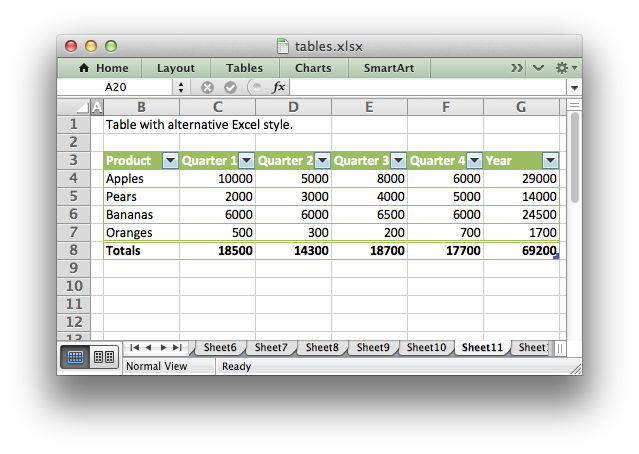The default table style is ‘Table Style Medium 9’.

You can also turn the table style off by setting it to None:

```worksheet.add_table('B3:F7', {'data': data, 'style': None})
```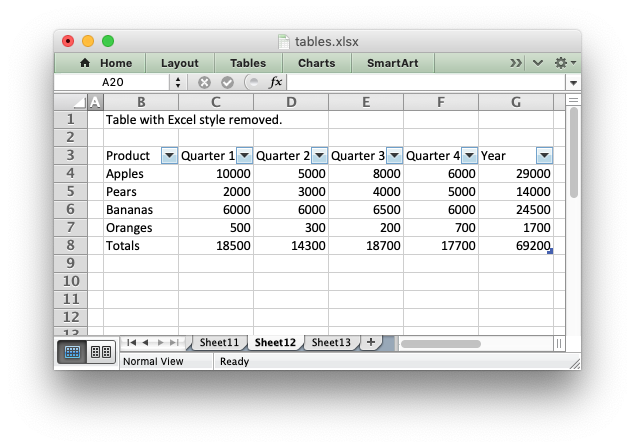## name

By default tables are named `Table1`, `Table2`, etc. The `name` parameter can be used to set the name of the table:

```worksheet.add_table('B3:F7', {'name': 'SalesData'})
```

If you override the table name you must ensure that it doesn’t clash with an existing table name and that it follows Excel’s requirements for table names, see the Microsoft Office documentation.

If you need to know the name of the table, for example to use it in a formula, you can get it as follows:

```table = worksheet.add_table('B3:F7')
table_name = table.name
```

## total_row

The `total_row` parameter can be used to turn on the total row in the last row of a table. It is distinguished from the other rows by a different formatting and also with dropdown `SUBTOTAL` functions:

```worksheet.add_table('B3:F7', {'total_row': True})
```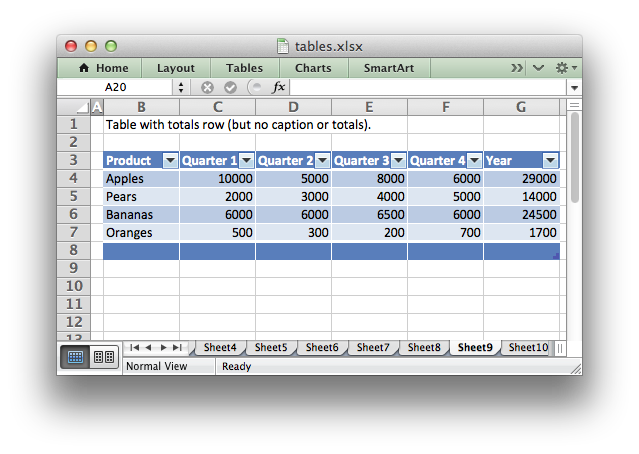The default total row doesn’t have any captions or functions. These must by specified via the `columns` parameter below.

## columns

The `columns` parameter can be used to set properties for columns within the table.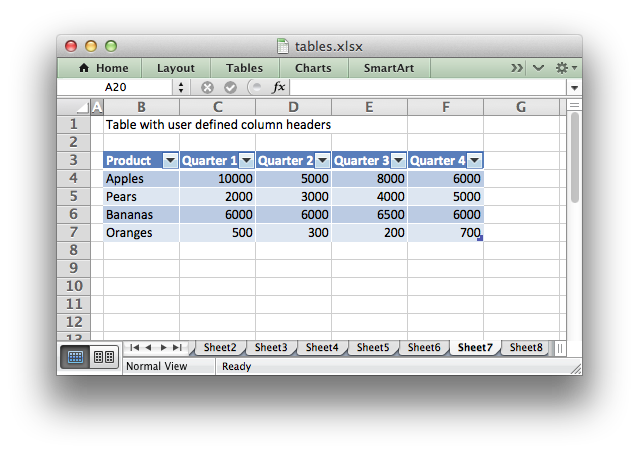The sub-properties that can be set are:

The column data must be specified as a list of dicts. For example to override the default ‘Column n’ style table headers:

```worksheet.add_table('B3:F7', {'data': data,
]})
```

See the resulting image above.

If you don’t wish to specify properties for a specific column you pass an empty hash ref and the defaults will be applied:

```...
columns, [
{},                     # Defaults to 'Column 3'.
]
...
```

Column formulas can by applied using the column `formula` property:

```formula = '=SUM(Table8[@[Quarter 1]:[Quarter 4]])'

'formula': formula},
]})
```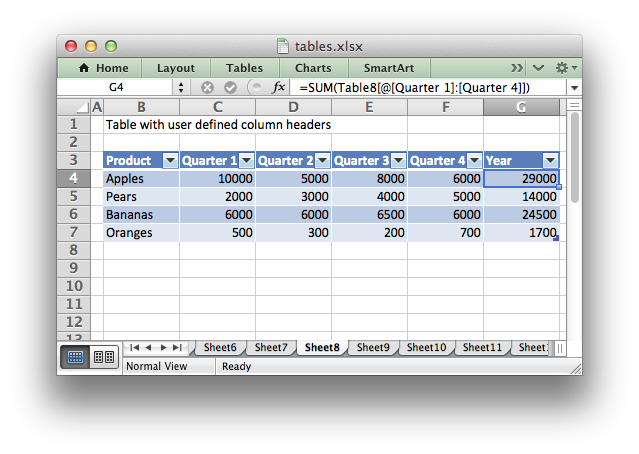The Excel 2007 style `[#This Row]` and Excel 2010 style `@` structural references are supported within the formula. However, other Excel 2010 additions to structural references aren’t supported and formulas should conform to Excel 2007 style formulas. See the Microsoft documentation on Using structured references with Excel tables for details.

As stated above the `total_row` table parameter turns on the “Total” row in the table but it doesn’t populate it with any defaults. Total captions and functions must be specified via the `columns` property and the `total_string` and `total_function` sub properties:

```options = {'data': data,
'total_row': 1,
'formula': '=SUM(Table10[@[Quarter 1]:[Quarter 4]])',
'total_function': 'sum'
},
]}

# Add a table to the worksheet.
```

The supported totals row `SUBTOTAL` functions are:

 average count_nums count max min std_dev sum var

User defined functions or formulas aren’t supported.

It is also possible to set a calculated value for the `total_function` using the `total_value` sub property. This is only necessary when creating workbooks for applications that cannot calculate the value of formulas automatically. This is similar to setting the `value` optional property in `write_formula()`:

```options = {'data': data,
'total_row': 1,
'columns': [{'total_string': 'Totals'},
{'total_function': 'sum', 'total_value': 150},
{'total_function': 'sum', 'total_value': 200},
{'total_function': 'sum', 'total_value': 333},
{'total_function': 'sum', 'total_value': 124},
{'formula': '=SUM(Table10[@[Quarter 1]:[Quarter 4]])',
'total_function': 'sum',
'total_value': 807}]}
```

Formatting can also be applied to columns, to the column data using `format` and to the header using `header_format`:

```currency_format = workbook.add_format({'num_format': '\$#,##0'})

'total_row': 1,
'total_function': 'sum',
'format': currency_format},